# INDEX & MATCH in Excel – a better alternative to VLOOKUP

0
5607This tutorial demonstrates the key strengths of Excel’s INDEX / MATCH function that make it superior to VLOOKUP. You will find a number of formula examples that will help you easily cope with many complex tasks when VLOOKUP fails.

In a couple of recent articles I made a good effort to explain the basics of Excel VLOOKUP functionto beginners and provide more complex VLOOKUP formula examples to power users. And now, I will try if not to talk you out of using VLOOKUP, then at least show you an alternative way to do a vertical lookup in Excel.

“What do I need that for?” you can ask me. Because VLOOKUP is not the only lookup formula available in Excel, and its numerous limitations might prevent you from getting the desired result in many situations. On the other hand, Excel’s INDEX MATCH is more flexible and has certain features that make it superior to VLOOKUP in many respects.## Excel’s INDEX and MATCH functions – the basics

Since the aim of this tutorial is to demonstrate an alternative way to do a vlookup in Excel by using a combination of INDEX and MATCH functions, we won’t dwell much on their syntax and uses. We will cover only the minimum necessary for understanding the general idea and then take an in-depth look at formula examples that reveal all the advantages of using Index / Match instead of Vlookup.

#### The INDEX function’s syntax and usage

The Excel INDEX function returns a value in an array based on the row and column numbers you specify. The syntax of the INDEX function is straightforward:

INDEX(array, row_num, [column_num])

Here is a very simple explanation of each parameter:

• array – this is a range of cells that you want to return a value from.
• row_num – the row number in array from which you want to return a value. If omitted, the column_num is required.
• column_num – the column number in array from which you want to return a value. If omitted, row_num is required.

If both row_num and column_num parameters are used, the INDEX function returns the value in the cell at the intersection of the specified row and column.

And here is the simplest example of the INDEX formula:

`=INDEX(A1:C10,2,3)`

The formula searches in cells A1 through C10 and returns a value of the cell in the 2nd row and the 3rd column, i.e. cell C2.

Very easy, right? However, when working with real data you would hardly ever know which row and column you want, that is why you need the help of the MATCH function.

#### The MATCH function’s syntax and usage

The Excel MATCH function searches for a lookup value in a range of cells, and returns the relative position of that value in the range.

For example, if the range B1:B3 contains the values “New-York”, “Paris”, “London”, then the formula `=MATCH("London",B1:B3,0)` returns the number 3, because “London” is the third entry in the range.

The syntax of the MATCH function is as follows:

MATCH(lookup_value, lookup_array, [match_type])
• lookup_value – this is the number or text you are looking for. This can be a value, a cell reference or logical value.
• lookup_array – a range of cells being searched.
• match_type – this parameter tells the MATCH function whether you want to return an exact match or the nearest match:
• 1 or omitted – finds the largest value that is less than or equal to the lookup value. The values in the lookup array must be sorted in ascending order, i.e. from smallest to largest.
• 0 – finds the first value that is exactly equal to the lookup value. In the INDEX / MATCH combination, you almost always need the exact match, so the third argument of your MATCH function is “0”.
• -1 – finds the smallest value that is greater than or equal to lookup_value. The values in the lookup array must be sorted in descending order, i.e. from largest to smallest.

At first sight, the usefulness of the MATCH function may seem questionable. Who cares about the position of a value in a range? What we do want to know is the value itself.

Let me remind you that the relative position of the lookup value (i.e. a row or/and column number) is exactly what you need to supply to the row_num or/and column_num argument of the INDEX function. As you remember, the INDEX function can return the value at the juncture of a given row and column, but it cannot determine which exactly row and column you want.

## How to use INDEX MATCH function in Excel

Now that you know the basics of these two functions, I believe it has already started making sense how Excel’s MATCH and INDEX work together.

The MATCH function determines the relative position of the lookup value in the specified range of cells. From there, the INDEX function takes that number, or numbers, and returns a value in the corresponding cell.

Still having difficulties to figure it out? Think about Excel INDEX / MATCH in this way:

INDEX (column to return a value from, MATCH (lookup valuecolumn to look up against, 0))

I believe it’s even easier to understand from an example. Suppose you have a list of national capitals like this: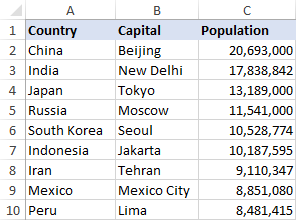Let’s find the population of some capital, say the capital of Japan, using the following Index Match formula:

`=INDEX(\$D\$2:\$D\$10,MATCH("Japan",\$B\$2:\$B\$10,0))`

Now, let’s analyze what each component of this formula actually does:

• The MATCH function searches for the lookup value “Japan” in column B, more precisely cells B2:B10, and returns the number 3, because “Japan” is the third in the list.
• The INDEX functions takes “3” in the second parameter (row_num), which indicates which row you want to return a value from, and turns into a simple `=INDEX(\$D\$2:\$D\$10,3)`. Translated into plain English, the formula reads: search in cells D2 through D10 and return a value of the cell in the 3rd row, i.e. cell D4, because we start counting from the second row.

And here’s the result you get in Excel: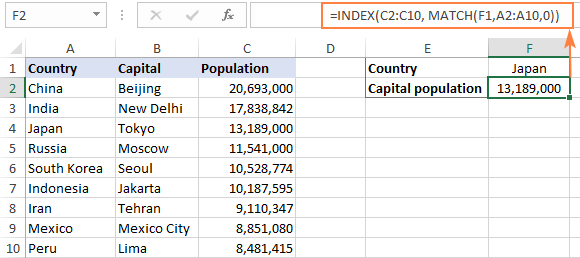Important! The number of rows and columns in the INDEX array should match those in the row_num or/and column_num parameters of the MATCH functions, respectively. Otherwise, the formula will return incorrect result.

Wait, wait… why don’t we simply use the following Vlookup formula? What’s the point in wasting time trying to figure out the arcane twists of Excel’s MATCH / INDEX?

`=VLOOKUP("Japan",\$B\$2:\$D\$2,3)`

In this case, no point at all : ) This simple example is for demonstration purposes only, so that you get a feel of how the Index and Match functions work together. Other examples that follow below will show you the real power of the INDEX MATCH liaison that easily copes with many complex scenarios when VLOOKUP stumbles.

## Why Excel’s INDEX MATCH is better than VLOOKUP

When deciding which formula to use for vertical lookups, the majority of Excel gurus agree that INDEX / MATCH is far better than VLOOKUP. However, many Excel users still resort to utilizing VLOOKUP because it’s a simpler function. This happens because very few people fully understand all the benefits of switching from Vlookup to Index Match, and without such understanding no one is willing to invest their time to learn a more complex formula.

Below, I will try to point out the key advantages of using MATCH / INDEX in Excel, and then you decide whether you’d rather stick with Vlookup or switch to Index Match.

#### 4 top benefits of using MATCH INDEX in Excel

1. Right to left lookup. As any educated user knows, Excel VLOOKUP cannot look to its left, meaning that your lookup value should always reside in the left-most column of the lookup range. INDEX MATCH can do a left lookup with ease! The following example demonstrates this feature in action – How to vlookup a value to the left in Excel.
2. Insert or delete columns safely. VLOOKUP formulas get broken or return incorrect results when a new column is deleted from or added to a lookup table. With VLOOKUP, any inserted or deleted column changes the results returned by your formulas because the VLOOKUP function’s syntax requires specifying the entire table array and a certain number indicating which column you want to pull the data from.For example, if you have a table A1:C10 and want to return a value from column B, you will put “2” in the third parameter (col_index_num) of a VLOOKUP formula, say `=VLOOKUP("lookup value", A1:C10, 2)`. If at a later point, you insert a new column between A and B, you will have to change “2” to “3” in your formula, otherwise it would return a value from the newly inserted column.

With INDEX MATCH, you can delete or insert new columns in your lookup table without distorting the results since you specify directly the column containing the value you want to get. And this is a really great benefit, especially when working with large datasets, since you are able to insert and remove columns without worrying about updating every associated vlookup formula.

3. No limit for a lookup value’s size. When using the VLOOKUP function, remember that the total length of your lookup criteria should not exceed 255 characters, otherwise you will end up having the #VALUE! error. So, if your dataset contains long strings, INDEX MATCH is the only working solution.Suppose, you are using the following VLOOKUP formula that searches through cells B5 to D10 for the value in cell A2:

`=VLOOKUP(A2,B5:D10,3,FALSE)`

The formula won’t work if the lookup value in cell A2 exceeds 255 symbols. Instead, you shall use the analogous INDEX / MATCH function:

`=INDEX(D5:D10, MATCH(TRUE, INDEX(B5:B10=A2, 0), 0))`

4. Higher processing speed. If your tables are relatively small, there will hardly be any significant difference in Excel’s performance. But if your worksheets contain hundreds or thousands of rows, and consequently hundreds or thousands of formulas, MATCH INDEX will work much faster than VLOOKUP because Excel will have to process only the lookup and return columns rather than the entire table array.VLOOKUP’s impact on Excel’s performance may be especially noticeable if your workbook contains complex array formulas like VLOOKUP and SUM. The point is that checking each value in the array requires a separate call of the VLOOKUP function. So, the more values your array contains and the more array formulas you have in a workbook, the slower Excel performs.

On the other hand, with INDEX MATCH, Excel has to consider only the lookup and return columns, as the result it processes such formulas much faster.

## Excel INDEX & MATCH – formula examples

Now that you know the reasons to learn the MATCH INDEX function, let’s get to the most interesting part and see how you can apply the theoretical knowledge in practice.

### How to look up from right to left with INDEX & MATCH

As stated in any VLOOKUP tutorial, this Excel function cannot look at its left. So, unless your lookup column is the left-most column in the lookup range, there’s no chance that a vlookup formula will return the result you want.

Excel’s INDEX MATCH function is more flexible and does not really care where the return column resides. As an example, we will use the table listing national capitals by population again. This time, let’s write an INDEX MATCH formula that finds how the Russian capital, Moscow, ranks in terms of population.

As you can see in the screenshot below, the following formula has not problem with performing a left vlookup:

`=INDEX(\$A\$2:\$A\$10,MATCH("Russia",\$B\$2:\$B\$10,0))`

Naturally, you can replace the “hard-coded” lookup value with a cell reference:

`=INDEX(\$A\$2:\$A\$10,MATCH(F2,\$B\$2:\$B\$10,0))`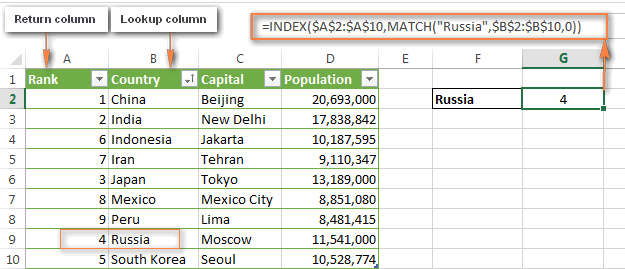By now, you should not have any difficulties to understand how the formula works:

• First, you write a simple MATCH formula that finds the position of Russia: `=MATCH("Russia",\$B\$2:\$B\$10,0))`
• Then, you determine the array parameter for your Index function, which is column A in our case (A2:A10).
• Finally, you assemble the two parts together and get this formula: `=INDEX(\$A\$2:\$A\$10,MATCH("Russia",\$B\$2:\$B\$10,0))`
Tip. It’s a good idea to always use absolute cell references in INDEX and MATCH formulas so that your lookup ranges won’t get distorted when you copy the formula to other cells.

### Calculations with INDEX MATCH in Excel (AVERAGE, MAX, MIN)

You can nest other Excel functions within the MATCH INDEX formula, say, to find the minimum or maximum value, or the value closest to the average in the range. Here are a few formula examples for the table used in the previous sample:

 Function Formula example Description Returned result Min =INDEX(\$C\$2:\$C\$10, MATCH(MIN(\$D\$2:I\$10), \$D\$2:D\$10, 0)) Finds the min value in column D and returns a value from column C in the same row. Beijing Max =INDEX(\$C\$2:\$C\$10, MATCH(MAX(\$D\$2:I\$10), \$D\$2:D\$10, 0)) Finds the max value in column D and returns a value from column C in the same row. Lima Average =INDEX(\$C\$2:\$C\$10, MATCH(AVERAGE(\$D\$2:D\$10), \$D\$2:D\$10, 1)) Calculates the average in range D2:D10, finds the value closest to the average, and returns a corresponding value from column C. Moscow

#### Things to keep in mind when using AVERAGE with INDEX / MATCH

When using the AVERAGE function in combination with INDEX MATCH, most often you would enter either “1” or “-1” in the third argument (match_type) of the MATCH function, unless you are certain that your lookup array contains a value exactly equal to the average. If latter, you can enter “0” for exact match.

If you set 1, the values in the lookup column must be sorted in ascending order, and the formula will return the largest value that is less than or equal to the average value.

If you set -1, the values in the lookup column must be sorted in descending order, and the smallest value that is greater than or equal to the average value will be returned.

In our example, values in column D are sorted in ascending order, so we use “1” as the match type, and our Average + Index Match formula returns “Moscow” since its population (11,5410,00) is the closest “less than” match to the average number (12,269,006).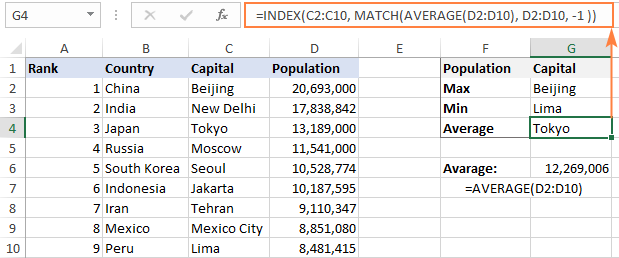If you are curious to know analogues VLOOKUP formulas, here you go: Calculations with VLOOKUP (AVERAGE, MAX, MIN).

### How to use INDEX MATCH to search by row and column values

This formula is an equivalent of the two-dimensional VLOOKUP that lets you find the value at the intersection of a certain row and column.

In this example, your Excel INDEX MATCH function is going to be very similar to other formulas we’ve already discussed in this tutorial, with the only difference. Guess what?

As you remember, the syntax of the INDEX function allows for both: `INDEX(array, row_num, [column_num])`. And I congratulate those of you who have guessed right : )

To begin with, let’s create the general formula to perform a matrix lookup. We just take the INDEX / MATCH formula you already know and add one more MATCH function to it, which will return the column number:

=INDEX (lookup table, MATCH (vertical lookup valuecolumn to look up against, 0), MATCH (horizontal lookup valuerow to lookup against, 0))

Please pay attention that you have to specify the entire table in the array argument of the INDEX function in case of two-way lookup.

And now, let us apply this formula template in practice. Below, you see a list of the most populated counties in the world. Suppose, you want to know the number of population in the USA in the year 2015: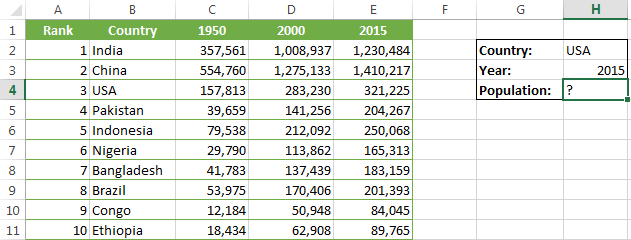Okay, let’s start on the formula. Whenever I need to create a complex Excel formula with one or several nested functions, I always write each individual function first.

So, you start by writing two MATCH functions that will return the row and column numbers for your INDEX function.

• Vertical match – you search through column B, more precisely in cells B2 to B11, for the value in cell H2 (“USA”), and the corresponding MATCH function is this:`=MATCH(\$H\$2,\$B\$1:\$B\$11,0)` This MATCH formula returns 4 because “USA” is the 4th item in column B (including the column header).
• Horizontal match – you search for the value in cell H3 (“2015”) in row 1, i.e. in cells A1 to E1: `=MATCH(\$H\$3,\$A\$1:\$E\$1,0)` This MATCH formula returns “5” because “2015” is the 5thcolumn.

Now, put the above formulas inside the INDEX function, and voila:

`=INDEX(\$A\$1:\$E\$11, MATCH(\$H\$2,\$B\$1:\$B\$11,0), MATCH(\$H\$3,\$A\$1:\$E\$1,0))`

If you replace the MATCH functions with the returned numbers, the formula is much easier to understand: `=INDEX(\$A\$1:\$E\$11, 4, 5, 0))`

Meaning, it returns a value at the intersection of the 4th row and 5th column in range A1:E11, which is the value in cell E4. Easy? Yep! : )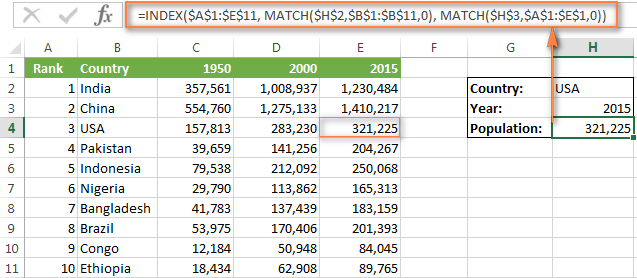### Look up with multiple criteria using INDEX MATCH

In the Excel VLOOKUP tutorial, I demonstrated a formula example to do a vlookup with multiple criteria. However, a significant limitation of that approach was the necessity to add a helper column. The good news is that Excel’s INDEX MATCH function can also look up values in 2 columns, at that no helper column is requited!

Suppose, you have a list of orders and you want to find the sum based on 2 criteria, “Customer Name” and “Product“. A complicating factor is that one customer can buy multiple products and customer names are listed in a random order in the Lookup table: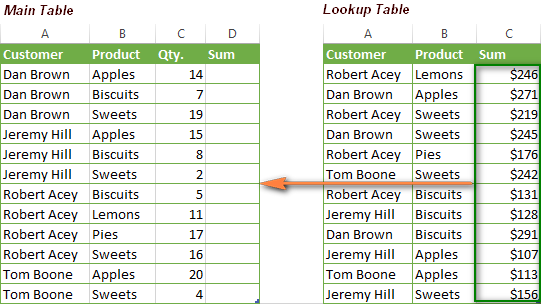The following INDEX MATCH array formula works a treat:

`=INDEX('Lookup table'!\$A\$2:\$C\$13, MATCH(1, (A2='Lookup table'!\$A\$2:\$A\$13) * (B2='Lookup table'!\$B\$2:\$B\$13) ,0), 3)`

This formula is more complex than any other we’ve discussed today, but powered with the knowledge of Excel MATCH INDEX you already have, I am sure you can cope with it.

The trickiest part is the MATCH function, so let’s figure it out first:

`MATCH(1, (A2='Lookup table'!\$A\$2:\$A\$13),0) * (B2='Lookup table'!\$B\$2:\$B\$13), 0)`

What we have here is the following 3 arguments:

• Lookup_value: 1
• Lookup_array: (A2=’Lookup table’!\$A\$2:\$A\$13),0) * (B2=’Lookup table’!\$B\$2:\$B\$13)
• Match_type: 0

The 1st and 3rd parameters are crystal clear – the function searches for “1”, and returns the first found value.

Now, the main question is – why do we look up “1”? To get the answer, let’s have a closer look at our lookup array.

What we do here is take the first value (A2) in the “Customer name” column in the main table and check it against all customer names in the lookup table (A2:A13). If the match is found, the equation returns TRUE, otherwise FALSE. Then we do the same for values in columns B (“Products”).

To better understand what I am talking about, you can select the arrays in the formula bar, and press the F9 key to see what each of the selected parts evaluates to (please see Evaluating portions of an array formula for more details):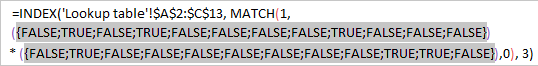As you probably know, in Excel formulas, the logical value of TRUE equates to 1, and FALSE equates to 0. And because the asterisk (*) acts as the AND operator in array formulas, we get “1” only if the match is found in both columns, 0 otherwise. So, the result of this operation is an array of 1’s and 0’s, where “1” is the value that meets both of the specified conditions. If your lookup table does not have any duplicated rows, there will be just one “1” in the array. And because “1” is our lookup value, the MATCH function returns the relative position of that row as demonstrated in the following screenshot: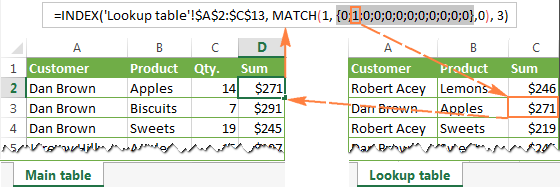Also, please pay attention that you have to use the optional third parameter (column_num) of the INDEX function. This is because you specify the entire table in the first parameter (array), and you need to let the function know which column you want to return a value from. In our case it’s column C (“Sum”), so we enter 3 in the third argument of the Index function.

And finally, since we need to check each cell in the array, our INDEX MATCH formula should be an array formula. The most obvious visual indication of an array formula is {curly braces} in which it is encapsulated in the formula bar. Just remember to press Ctrl+Shift+Enter to properly complete the formula after you’ve finished typing.

If everything is done correctly, you will get a result similar to what you see in the screenshot below: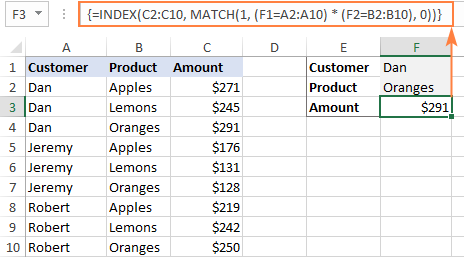### Using INDEX / MATCH with IFERROR in Excel

As you have probably noticed (more than once : ) if you enter an invalid value, i.e. a value that does not exist in the lookup array, the INDEX / MATCH function produces #N/A or #VALUE error message. If you’d rather replace it will something more meaningful, you can wrap your INDEX / MATCH formula in the IFERROR function.

The syntax of the IFERROR function is very simple:

IFERROR(value, value_if_error)

Where the value argument is the value checked for an error (the result of the INDEX MATCH formula in our case); and value_if_error is the value to return if the formula produces an error.

For example, you can enclose the formula from the previous example within the IFERROR function in this way:

`=IFERROR(INDEX(\$A\$1:\$E\$11, MATCH(\$G\$2,\$B\$1:\$B\$11,0), MATCH(\$G\$3,\$A\$1:\$E\$1,0)),`

“No match is found. Please try again!”)

And now, if someone inputs an invalid entry, the formula will produce the result you see in the screenshot below: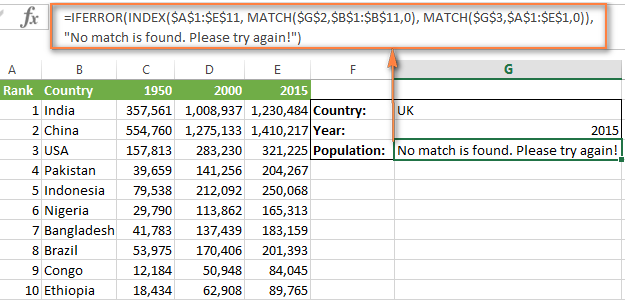If you prefer to have a blank cell when an error is returned, you can just use double quotes (“”) in IFERROR’s second parameter, like this:

IFERROR(INDEX(array, MATCH(lookup_value, lookup_array, 0), “”)